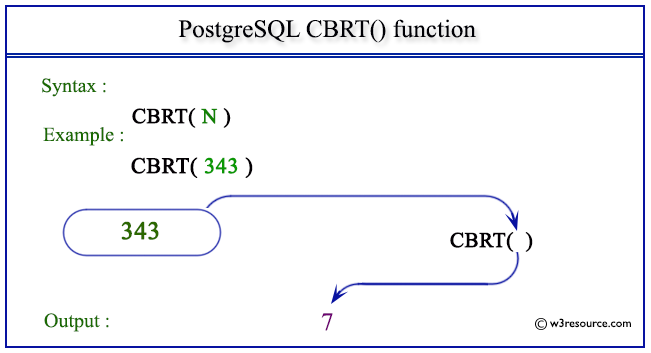# PostgreSQL CBRT() function

## CBRT() function

The PostgreSQL cbrt() function is used to return the cube root of a given number.

Syntax:

```cbrt()
```

PostgreSQL Version: 9.3

Pictorial presentation of PostgreSQL CBRT() functionExample: PostgreSQL CBRT() function

Code:

``````SELECT CBRT(343) AS "Cube Root";
```
```

Sample Output:

``` Cube Root
-----------
7
(1 row)
```

Example: PostgreSQL CBRT() function using negative value

Code:

``````SELECT CBRT(-343) AS "Cube Root";
```
```

Sample Output:

``` Cube Root
-----------
-7
(1 row)
```

Previous: ATAN2 function
Next: CEIL function

﻿